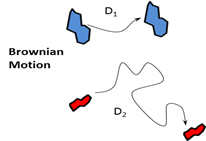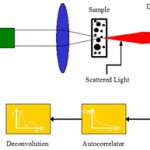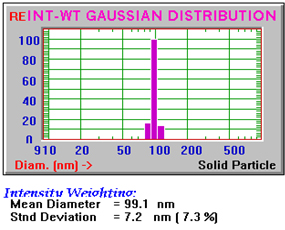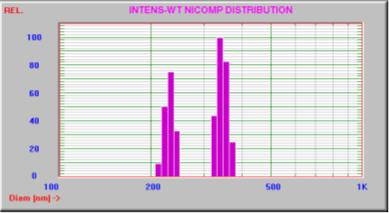• Cart
• LanguageChoose Language
• CurrencyChoose Currency

[latexpage]Dynamic Light Scattering (DLS) is also known as Photon Correlation Spectroscopy (PCS). DLS is used to size particles from below 5 nm to several microns. This technique operates on the principle that particles move randomly in gas or liquid i.e. undergo Brownian motion. The movement (diffusion) of these particles is described by the Stokes-Einstein equation (Eq.1).

$D = k_BT/6\pi\eta R$ (Eq. 1)

Where the diffusion (D) is equal to the product of Boltzman’s constant (k_$B$) divided by the hydrodynamic radius of the particle (R) of the particle and the shear viscosity of the solvent ($\eta$). Larger particles have a slower velocity and will have smaller coefficients of diffusion than larger particles.

In most DLS systems a laser (i.e. HeNe) of known wavelength passes through a dilute sample in solution and the intensity of scattered light is collected by a detector and deconvoluted by algorithms to determine the particle size distribution of the sample. Figure B shows a schematic of a DLS instrument.The amount of scattered light collected is dependent on the molecular weight, size, and shape of a particle as well as the refractive indices of the particle and solvent. Before reaching the detector, the scattered light from individual particles experiences interference from those scattered by other particles all of which are moving randomly due to Brownian motion. This results in random fluctuations in time.Figure C shows a typical intensity vs time plot for three differently sized particles diffusing in solution. The time scale of the fluctuations shown in the figure is dependent on the particle diffusivity and size of the particles. Smaller particles will jitter about more quickly than larger particles. The figure shows represenative time vs intensity plots for “small” (3a), “medium” (3b) and “large” (3c) particles.

To determine the numerical size of the particles it is necessary to correlate intensity to the diffusion coefficient of the particles. This is done using an autocorrelation function or ACF (eq. 2). This function examines the changes in scattered intensity over periods of time for a volume of particles. In the case of a simple monodisperse particle size distribution (PSD) the ACF is a single decaying exponential function (eq. 3). After a series of calculations a decay constant (tt) is found that is inversely proportional to the diffusivity of a particle as shown in equations 4(a-b) where K is a constant called the “scattering wave vector”. This constant relates the time scale of the diffusion process to the distance scale set by the laser wavelength. K is shown in equation 5 and is dependent on the wavelength of the laser (λl), q is the angle of detection and the index of refraction of the solvent (n). Once the coefficient of diffusion is known the hydrodynamic radius can be determined using the Stokes-Einstein equation (eq. 6).

$C(t’) = (eq. 2)$

$C(t') = A exp(-t'/\tau) + B (eq. 3)$

$1/\tau = 2DK^2 (eq. 4a)$

$D = (1/2K^2)(1/\tau) (eq. 4b)$

$K = (4\pi\eta/\lambda) sin \theta/2 (eq. 5)$

$D=kT/6\pi \eta R (eq. 6)$The simplest DLS result is of a monodisperse or uniform sample with a narrow size distribution. A monodisperse sample will have a bell shaped curve of narrow width (Figure D). Such a sample is quite rare. Most samples will have either a broad Gaussian single peak PSD indicating that the sample is not uniform and has perhaps a skewed unimodal or multimodal PSD. A simple Gaussian calculation will give highly inaccurate results.

In order to analyze samples that are not perfectly monodisperse, PSS employs a proprietary inverse Laplace transform called the NICOMP distribution analysis that does not assume a particular shape for the PSD and analyzes up to four independent parameters to determine PSDs. Figure E shows a PSD for a bimodal sample containing 220nm and 340nm polystyrene latex spheres. A conventional Gaussian analysis would not be able to discern the two mean diameters and would present a result with a mean diameter somewhere in between to the two.